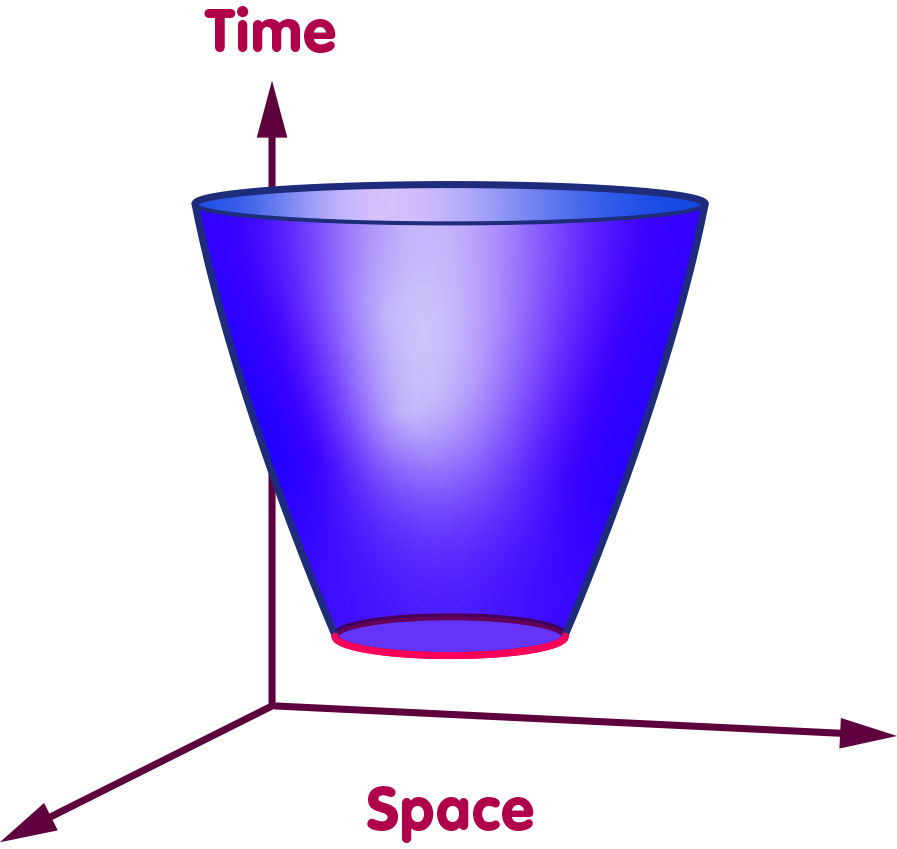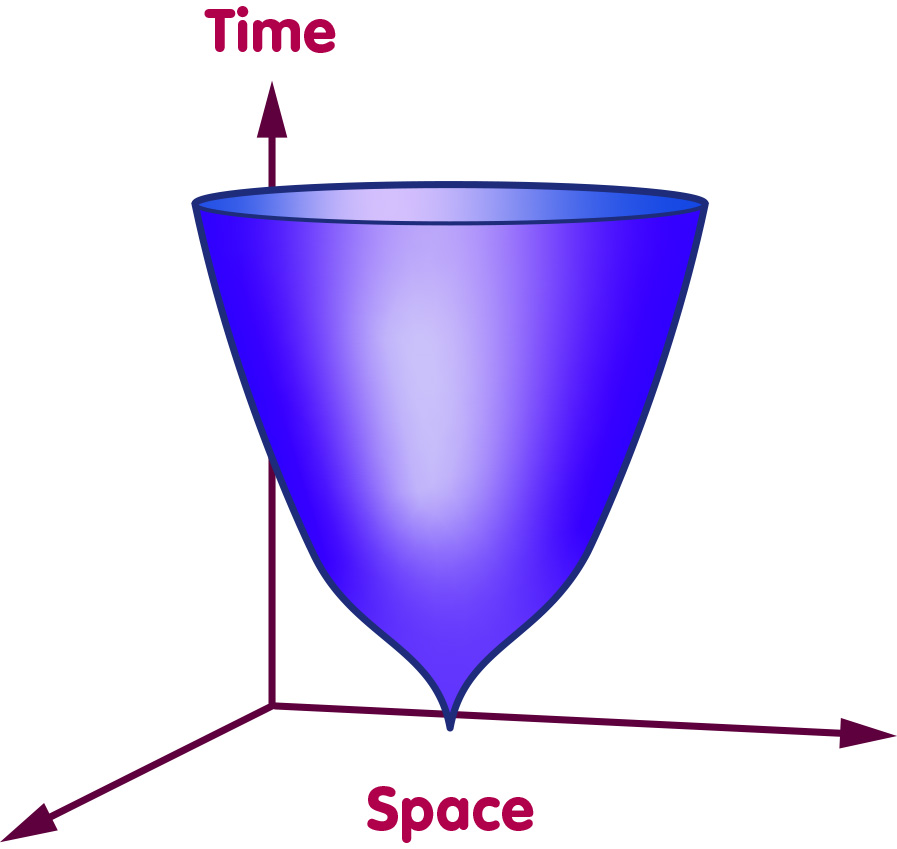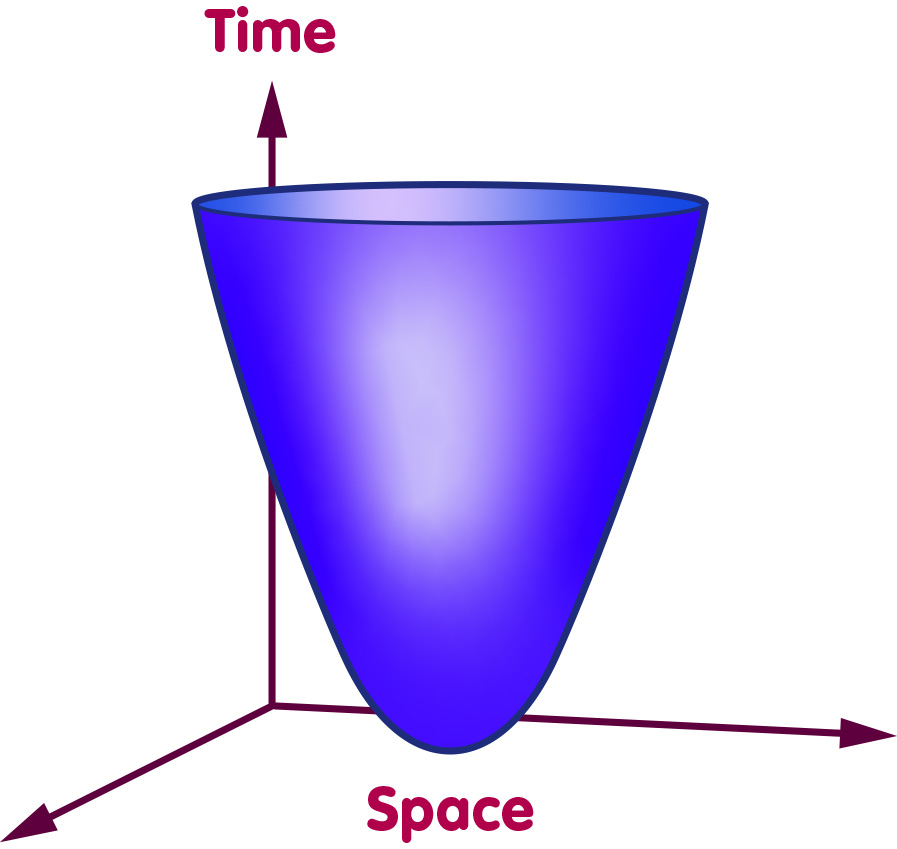# Searching for the quantum beginning of the universe

### About attempts to understand the beginning of our universe using different approaches to quantum gravity

#### An article by Jorma Louko

Suppose that we have at hand a quantum theory of all the forces of nature, including gravitation. What would we hope to do with this theory?

As one example, the theory should predict what happens in the innermost regions of a black hole formed by the collapse of a star – regions in which matter and energy are compressed to a density at which quantum gravity effects should become important. As a second example, the theory should predict what happens when elementary particles collide at energies so large that the quantum nature of spacetime plays a significant role in the collision dynamics. In some theories of quantum gravity this energy is within the reach of particle accelerators that are currently under construction, and the theories indeed predict how frequently miniature black hole pairs would be produced in the accelerators. In less optimistic theories the threshold energy is of the order of Planck energy, which is far beyond the capabilities of accelerators that could be built with current technology, but even in this case the theory should provide a prediction that a technologically vastly more advanced civilization could in principle test in their gigantic accelerators.

These examples have an important aspect in common. They start out with a situation that can be described without a theory of quantum gravity – an ordinary star, say, or two particles heading for a collision. Quantum gravity is needed only to describe how the situation then develops – how the collapsing star forms the interior of a black hole, or what happens in the high energy density region where the particles collide. To summarise: In these examples the initial conditions can be described in terms of classical spacetime, while a theory of quantum gravity is needed for predicting the subsequent evolution.

But what could a theory of quantum gravity predict about the earliest universe?

## The need for an initial quantum state

The classical big bang cosmological models describe gravitation by Einstein’s general theory of relativity. These models are able to treat the matter content of the universe quantum mechanically where appropriate, but they do not take into account the interplay of quantum theory and gravity. While the models have been successful in describing most of the history of our universe, they become ill-defined at the very beginning of the universe – at the big bang singularity. At this singularity, both the curvature of space and the density of matter are infinite – a state of affairs that is unacceptable for a physical theory.

It is widely expected that the infinities of the big bang singularity will no longer be present in a theory in which also the dynamics of spacetime is treated quantum mechanically. Here, however, the situation is fundamentally different from the two examples mentioned above. The earliest universe was presumably quantum mechanical through and through, and we can no longer describe its initial conditions in a classical language. We must instead treat the whole early universe as a quantum system and formulate its initial conditions in the language of quantum mechanics. We need to ask the basic question of quantum cosmology: What was the initial quantum state of the universe?

Quantum gravity theorists have not shied away from the challenge. At present there are some half a dozen contenders for a principle that would single out, from all the quantum states allowed by the dynamical laws of quantum gravity, the initial quantum state of our universe.

## A universe without a (past) boundary?

Perhaps most studied among the proposals for the initial state of our universe is the “no-boundary” proposal of James Hartle and Stephen Hawking, dating from 1983. To state this proposal, we first need to recall that in Feynman’s path-integral formulation of quantum mechanics, the probability of the universe to evolve from an initial state A to a later state B is calculated using a kind of sum over all conceivable ways this evolution could have taken place – over all possible histories leading from state A to state B. (For more information on path integrals, see the spotlight text The sum over all possibilities.) Now, Hartle and Hawking proposed a specific initial boundary condition for the histories that describe our universe. The gist of the proposal can be seen by considering a simplified model universe with only one space dimension, evolving over time from an initial state. We take space in this model universe to be shaped like a circle:If we plot the evolution of space over time, the circle traces out a sort of tubular, two-dimensional surface. The following picture shows one such surface, in which the universe begins in some initial state (the circular boundary at the earliest time, traced in red) and, over time, expands (reading from bottom to top as time progresses, the circles stacked to form the two-dimensional surface get ever larger):Another possibility would be a universe that starts at zero size, not smoothly, but with a kind of singular cusp, as pictured in the following image:Hartle and Hawking’s proposal is that the initial state should have zero size but the evolution from this state should start smoothly, without any singularities. In our model universe this proposal would mean that the geometry at the initial state may not have a sharp end, such as the tip of a cone, but must instead be smooth, as in the following picture:The boundary condition is thus that the spacetime histories have no initial boundary in the past. The only boundary that enters the path integral calculations is the boundary at the “present”, at the moment at which we make observations about the universe.

## Tunnelling or symmetry?

Another proposal focusses on the observation that matter density at the classical big bang singularity is infinite. This means that it is classically impossible for the universe to start from zero size with finite matter density. In quantum mechanics, however, systems can undergo evolution that is classically forbidden, via a phenomenon called tunnelling. Classically, an object that does not have enough energy to climb over a hill will not get to its other side. But a quantum mechanical object that does not have enough energy to climb over a hill can nevertheless suddenly disappear from one side and appear on the other, as if instantaneously burrowing through a tunnel. One example of tunnelling is the spontaneous radioactive decay of certain atomic nuclei. Applying this picture to the beginning of the universe, one can thus envisage the universe as starting from zero size with finite matter density via a quantum tunnelling event. Implementations of this idea, known as the tunnelling proposal, have been advocated by Andrei Linde and Alexander Vilenkin.

Yet another proposal is based on the observation that the current rate of cosmological expansion appears to be the same in all spatial directions. While some cosmological models attribute this spatial large scale isotropy to dynamical evolution that has taken place since quantum gravity effects ceased to be important, a quantum gravity theorist would aspire to explain the isotropy directly by a quantum mechanical initial condition. This idea is implemented in the “symmetric initial condition” advocated by Heinz-Dieter Zeh, in which the zero volume singularity is postulated to have the maximum amount of isotropy compatible with Heisenberg’s uncertainty principle.

## Open problems

The quantum state of the universe is ultimately an experimental question and should be resolved by comparison with observation. The ‘correct’ quantum state should at the very least be compatible with our present-day cosmological data, including the large-scale homogeneity and isotropy and the small observed anisotropies in the cosmic microwave background. Computing classical predictions of this sort from any proposed initial quantum state remains however a formidable challenge. There are two reasons for this. The first is technical, the second conceptual.

The technical reason is that making predictions from a given initial quantum state requires a sufficiently good control of the dynamical laws of quantum gravity. With our current candidates for a quantum theory of gravity, such a control exists if the theory is first classically specialised to a cosmological model universe that only incorporates a few parameters to describe the universe. For example, a homogeneous model universe sets the matter density to be the same at every point in space, describing thus the matter distribution by just one parameter, the average density. It takes however an infinite number of parameters to describe the real universe: The matter density in the real universe is not the same at every point in space. It is currently not well understood how accurately the quantum dynamics of quantised cosmological models, with only a few parameters, approximate the quantum dynamics of the real universe, with its infinite number of parameters.

The conceptual reason goes right to the heart of quantum theory. In the overwhelming majority of applications of quantum theory, physicists are dealing with a comparatively small quantum system on the one hand (a molecule, or colliding elementary particles), and on the other hand with measuring devices (such as particle detectors) that can be understood largely without resorting to quantum physics. The standard ways in which physicists extract predictions from quantum theory make use of this two-fold view of the world – here the quantum system, there the measuring device. In quantum cosmology, where the quantum system is the whole universe, this standard view is not valid any more. What is required is a working conceptual understanding of how to make classical predictions from a quantum state when even the observers are part of the quantum system that they observe. In the pursuit of this understanding, quantum cosmologists have in the past two decades found much common ground with laboratory quantum physicists whose experiments can in certain circumstances exhibit quantum mechanical behaviour between two ends of a room, or in some recent experiments even between the two banks of the Danube.

## Further Information

For the basic concepts of relativity of interest for this spotlight topic, check out Elementary Einstein, in particular the chapters Relativity and the quantum and Cosmology.

Related Spotlights on relativity on Einstein-Online can be found in the sections Relativity and the quantum and Cosmology.

###### Colophon
Jorma Louko

is an Associate Professor of Mathematics at the University of Nottingham. His main research interest is quantum gravity, including quantum cosmology.